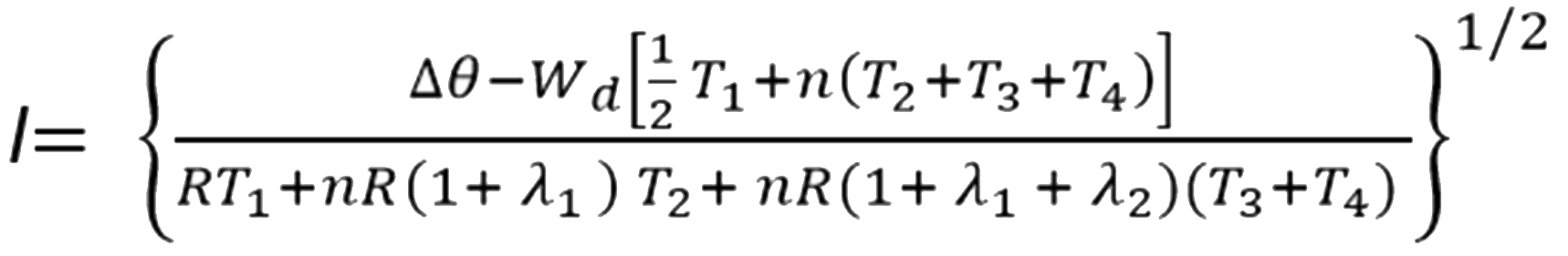# How is current carrying capacity calculated?

The current carrying capacity of an insulated conductor or cable is the maximum current (number of ampheres) that it can continuously carry without exceeding its temperature rating. It is also known as ampacity.

Whilst the cables are in operation they suffer electrical losses which manifest as heat in the conductor, insulation and any other metallic components in the construction. The current rating will depend on how this heat is dissipated through the cable surface and into the surrounding areas. The temperature rating of the cable is a determining factor in the current carrying capacity of the cable. The maximum temperature rating for the cable is essentially determined by the insulation material.

By choosing an ambient temperature as a base for the surroundings, a permissible temperature rise is available from which a maximum cable rating can be calculated for a particular environment. If the thermal resistivity values are known for the layers of materials in the cable construction then the current ratings can be calculated.

The formula for calculating current carrying capacity is:I = permissible current rating

∆Φ = Conductor temperature rise in (K)

R= Alternating current resistance per unit length of the conductor at maximum operating temperature (Ω/m)

Wd = dielectric loss per unit length for the insulation surrounding the conductor (W/m)

T1= Thermal resistance per unit length between one conductor and the sheath (K m/W)

T2 = thermal resistance per unit length of the bedding between sheath and the armour (K m/W)

T3 = thermal resistance per unit length of the external Sheath of the cable (K m/W)

T4 = thermal resistance per unit length between the cable surface and the surrounding medium (K m/W)

n = number of load-carrying conductors in the cable (conductors of equal size and carrying the same load)

λ1 = Ratio of losses in the metal sheath to total losses in all conductors in that cable

λ2 = ratio of losses in the armouring to total losses in all conductors in that cable.#### Cable Portfolio

View our comprehensive range of power, data, control and instrumentation cables and accessories

Go#### Cable Testing

Read more on the different tests we conduct in our Cable Laboratory

the Tests#### Case Studies

Read about some of the projects we've worked on, spanning all industries